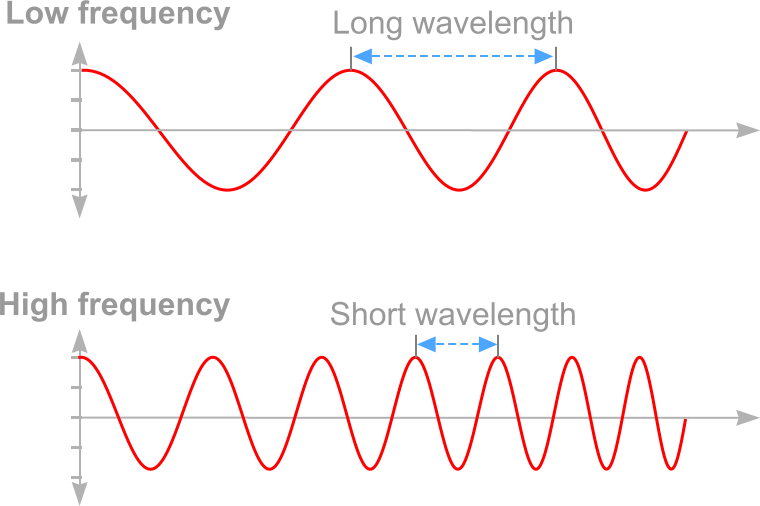The rate at which something occurs over a particular period of time. In the context of our fabrics, frequency describes the number of waves that pass a fixed point in a given amount of time.For example if the time it takes for a wave to pass is ½ second, the frequency is 2 per second. If it takes 1/100 of an hour, the frequency is 100 per hour.

Frequency is measured in Hertz (Hz) and is named after the German physicist Heinrich Hertz.
Frequency is the measure of 1 wave cycle per secod (1 Hz).

Units of measure

 1 Hz = 1 cycle per second 1000 Hz = 1 Kilohertz (kHz) 1000 kHz = 1 Megahertz (MHz) 1000 MHz = 1 Gigahertz (GHz) 1 GHz  =  1 Billion ( 1,000,000,000 Hz )